# An early fault feature extraction method for rolling bearings based on variational mode decomposition and random decrement technique

## Chengcheng Zhu1, Kaiming Teng2

2Rocket Army Material Purchasing Center, Beijing, China

2Corresponding author

Vibroengineering PROCEDIA, Vol. 18, 2018, p. 41-45. https://doi.org/10.21595/vp.2018.19930
Received 17 April 2018; accepted 28 April 2018; published 22 May 2018

32nd International Conference on Vibroengineering in Brno, Czech Republic, May 22-24th, 2018

Copyright © 2018 Chengcheng Zhu, et al. This is an open access article distributed under the Creative Commons Attribution License, which permits unrestricted use, distribution, and reproduction in any medium, provided the original work is properly cited.
Views 50
Abstract.

The early fault characteristics of rolling bearing are weak, and the background noise is so strong that it is difficult to diagnose. In order to solve the above problems, an early fault feature extraction method for rolling bearings based on variational mode decomposition and random decrement technique was proposed. The variational mode decomposition was used to decompose the collected vibration signals, and the component with the larger correlation coefficient was selected as the fault component. Then the fault component was processed by random decrement technology, and the Hilbert envelope spectrum of the fault component was made. According to the proposed method, the early fault characteristic of outer ring of rolling bearing was extracted. Compared with the method based on EMD, the proposed method is more effective in extracting the early fault characteristics of rolling bearings.

Keywords: variational mode decomposition, random decrement technique, rolling bearing, early fault feature extraction.

#### 1. Introduction

Rolling bearing is an important component in the mechanical system and the damage of rolling bearing will cause the failure of the mechanical system. It is of great significance to extract fault information and eliminate hidden dangers in the early stage of rolling bearing failure . Therefore, it is very important to diagnose the early faults of rolling bearings. In engineering applications, the early failure characteristics of bearings are rather weak. The vibration transmission path is very complex and the background noise is strong, making the feature extraction of early failure of rolling bearing becomes a difficult point .

Huang et al proposed the Empirical Mode Decomposition (EMD) . EMD has been widely used in early fault diagnosis of rolling bearings since it was put forward . EMD is a powerful tool for analyzing non-stationary signals and nonlinear signals. However, there is a lack of strict mathematical foundation, low algorithm efficiency and modal aliasing.

In 2014, Dragomiretskiy et al.  proposed a new adaptive signal processing method, Variational Mode Decomposition (VMD). In the process of obtaining the decomposed components, the method iteratively searches the optimal solution of the variational model to determine the frequency center and bandwidth of each component. Thus, the frequency division of signals and the effective separation of components can be realized adaptively. Compared with EMD, VMD has a solid theoretical foundation, and its essence is a number of adaptive Wiener filtering groups, showing better noise robustness . However, there is nothing VMD can do about the random noise in the signal.

In order to solve the problem, the random decrement technique (RDT) is introduced in this paper. Random decrement technique is a method of identification of modal parameters, which is first proposed by Cole [7, 8] in 70s. The basic concept of RDT is to assume a system with stationary random excitation, and its response is the superposition of both deterministic and random responses. The deterministic response is separated from the random response, and the random response is eliminated by using the statistical mean method. Finally, a deterministic free attenuation signal is obtained by filtering. RDT has been widely used in many fields such as vibration modal analysis , and structural damage detection .

Based on VMD and random decrement technique, a new early fault diagnosis method for rolling bearings is proposed in this paper. The method proposed in this paper is applied to early fault diagnosis of rolling bearings. The fault characteristics of rolling bearing are successfully extracted, and the practicability and effectiveness of the method are verified.

#### 2.1. Variational Mode Decomposition

According to the Reference , we get the complete algorithm for VMD, summarized in following algorithm.

Initialize$\left\{{\stackrel{^}{u}}_{k}^{1}\right\}$, $\left\{{\stackrel{^}{\omega }}_{k}^{1}\right\}$, $\left\{{\stackrel{^}{\lambda }}^{1}\right\}$, $n←0$

Repeat $n←n+1$

for$k=1:K$do

Update${\stackrel{^}{u}}_{k}^{n+1}$for all$w\ge 0$:

(1)
${\stackrel{^}{u}}_{k}^{n+1}\left(\omega \right)←\frac{\stackrel{^}{f}\left(\omega \right)-{\sum }_{i}{\stackrel{^}{u}}_{i}\left(\omega \right)+\frac{\stackrel{^}{\lambda }\left(\omega \right)}{2}}{1+2\alpha \left(\omega -{\omega }_{k}{\right)}^{2}},$

Update${\omega }_{k}$:

(2)
${\omega }_{k}^{n+1}\left(\omega \right)=\frac{{{\int }_{0}^{\infty }\omega \left|{\stackrel{^}{u}}_{k}\left(\omega \right)\right|}^{2}d\omega }{{{\int }_{0}^{\infty }\left|{\stackrel{^}{u}}_{k}\left(\omega \right)\right|}^{2}d\omega },$

end for

Dual ascent for all$\omega \ge 0$:

(3)
${\stackrel{^}{\lambda }}^{n+1}\left(\omega \right)←{\stackrel{^}{\lambda }}^{n}\left(\omega \right)+\beta \left[\stackrel{^}{f}\left(\omega \right){\sum }_{k=1}^{K}{\stackrel{^}{u}}_{k}^{n+1}\left(\omega \right)\right],$

until convergence:

(4)
$\frac{{{\sum }_{k=1}^{K}‖{{\stackrel{^}{u}}_{k}}^{n+1}-{{\stackrel{^}{u}}_{k}}^{n}‖}_{2}^{2}}{{‖{{\stackrel{^}{u}}_{k}}^{n}‖}_{2}^{2}}

The reconfiguration signal can be expressed as:

(5)
$\stackrel{^}{f}\left(t\right)={\sum }_{k=1}^{K}{\stackrel{^}{u}}_{k},$

#### 2.2. Random decrement technique

RDT can be used to describe the impulse response of the system, and its advantage is that it can extract free impact response from the stationary random response in the system. The core is to assume that a system is subjected to stationary random excitation, and the response is the superposition of deterministic response determined by the initial condition and random response determined by the initial external load. Under the same initial conditions, stationary random response is divided into several sections, and the general mean of the intercept segments is calculated, so as to extract the free attenuation response.

The response signal is divided into $L$ segments, and each segment of the signal is expressed as ${x}_{i}\left(t\right)$, whose length is $\tau$. Each response signal has the same trigger value, and the trigger value can be expressed as:

(6)
${x}_{i}\left({t}_{i}\right)={x}_{s}=const,i=1,2,\cdots ,L.$

The overall mean of the $L$ segment signal is calculated, and the random decrement function can be expressed as:

(7)

where ${x}_{i}\left({t}_{i}\right)={x}_{s},i=1,2,\cdots ,L$.

#### 2.3. Proposed method

The variational mode decomposition is used to decompose the collected vibration signals, and the component with the larger correlation coefficient is selected as the fault component. Then the fault component is processed by random decrement technology, and the Hilbert envelope spectrum of the fault component is made. According to the proposed method, the early fault characteristic of rolling bearing outer ring is extracted.

#### 3.1. Experiment condition

The experimental system is shown in Figure 1, including vibration sensor, coupling, driving motor, torque decoder/encoder and dynamometer. The type of the test bearing is SKF 6205-2RS, and the technical parameters of the bearing are shown in Table 1.

Fig. 1. The experimental system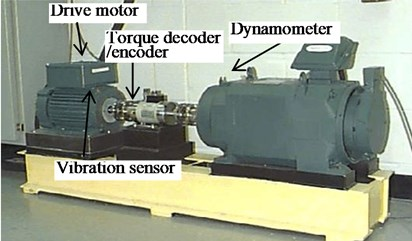Electric spark is used to process faults on bearing outer ring. The fault of diameter 0.18 mm and depth 0.28 mm is used to simulate the slight faults of outer ring. The speed of the driving motor is 1750 r/min, the sampling frequency is 12000 Hz, and the number of sampling points is 32768. Through theoretical calculation, the rotation frequency of the bearing can be obtained ${f}_{r}=$ 29.16 Hz; the fault characteristic frequency of the bearing outer ring is ${f}_{Oi}=$ 104.3Hz.

Table 1. The technical parameters of the bearing

 Internal diameter (${d}_{i}$) / mm Outside diameter (${d}_{o}$) / mm Roller diameter ($d$) / mm Pitch diameter ($D$) / mm Number of rolling element ($Y$) Contact angle$\alpha$ / (°) 25 52 8 39 9 0

#### 3.2. Experimental data processing

The time-domain and frequency domain waveform of the early fault vibration signal of the rolling bearing outer ring is shown in Fig. 2. It can be seen from Fig. 2 that the vibration signal has obvious impact components and there is a lot of background noise. The spectrum components are complex and can not extract the rotation frequency (29.16 Hz) and outer ring fault characteristic frequency (104.3 Hz).

Fig. 2. The time-domain and frequency domain waveform of the early fault vibration signal of the rolling bearing outer ring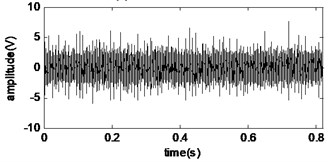a) Time domain waveform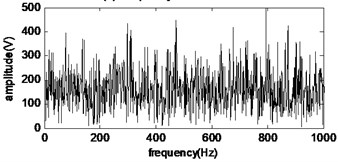b) Frequency domain waveform

In order to highlight the fault features, the vibration signal is processed by the method proposed in this paper. The number of decomposed modes $K$ is 4. The vibration signal is decomposed by VMD, and the component U2 and U3 with the largest correlation coefficient of vibration signal are selected as fault components. Then the fault component is processed by random decrement method and its Hilbert envelope spectrum is calculated, as shown in Fig. 3. It can be seen from Fig. 3 that the fault component of rolling bearing outer ring contains many characteristic frequencies, including 2 times the frequency 58.6 Hz, the outer ring fault characteristic frequency 100.1 Hz (the reason which is not exactly consistent with the theoretical value 104.3 Hz is the effect of the random sliding of the rolling element) and its 2 frequency doubling 200.2 Hz. In addition, there are also modulation sidebands, such as 158.7 Hz ($\text{2}{f}_{r}+{f}_{Oi}=$ 158.7 Hz), 258.8 Hz ($\text{2}{f}_{r}+\text{2}{f}_{Oi}=$ 258.8 Hz), 358.9 Hz ($\text{2}{f}_{r}+\text{3}{f}_{Oi}=$ 358.8 Hz), and 459 Hz ($\text{2}{f}_{r}+\text{4}{f}_{Oi}=$ 459 Hz). Therefore, the proposed method successfully extracted the characteristic frequency of the early fault of the outer ring of the rolling bearing.

Fig. 3. The Hilbert envelope spectrum of the fault component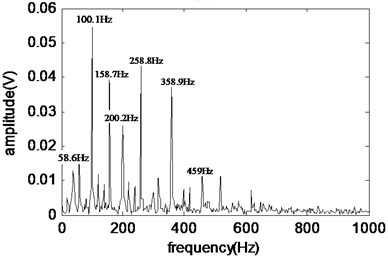a) U2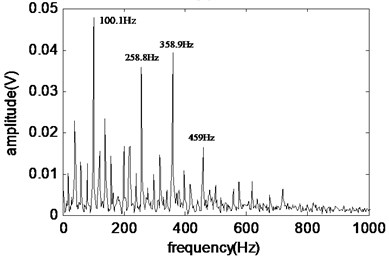b) U3

#### 4. Conclusions

In engineering applications, the early failure characteristics of bearings are rather weak. The vibration transmission path is very complex, and the background noise is strong, making the feature extraction of early failure of rolling bearing becomes a difficult point. A new method of rolling bearing fault feature extraction based on RDT and VMD is presented in this paper. At the same time, the research on early fault feature extraction of rolling bearing is carried out. The proposed method successfully extracted the characteristic frequency of the early fault of the outer ring of the rolling bearing.

1. Zhang Y., Randall R. B. Rolling element bearing fault diagnosis based on the combination of genetic algorithms and fast kurtogram. Mechanical Systems and Signal Processing, Vol. 23, Issue 5, 2009, p. 1509-1517. [Publisher]
2. Wang X., Zi Y., He Z. Multiwavelet denoising with improved neighboring coefficients for application on rolling bearing fault diagnosis. Mechanical Systems and Signal Processing, Vol. 25, Issue 1, 2011, p. 285-304. [Publisher]
3. Huang N. E., Shen Z., Long S. R. The empirical mode decomposition and the Hilbert spectrum for nonlinear and non-stationary time series analysis. Proceedings Mathematical Physical and Engineering Sciences, Vol. 454, Issue 1971, 1998, p. 903-995. [Publisher]
4. Yu D., Cheng J., Yang Y. Application of EMD method and Hilbert spectrum to the fault diagnosis of roller bearings. Mechanical Systems and Signal Processing, Vol. 19, Issue 2, 2005, p. 259-270. [Publisher]
5. Dragomiretskiy K., Zosso D. Variational mode decomposition. IEEE Transactions on Signal Processing, Vol. 62, Issue 3, 2013, p. 531-544. [Publisher]
6. Zhao C., Feng Z. P. Application of multi-domain sparse features for fault identification of planetary gearbox. Measurement, Vol. 104, 2017, p. 169-179. [Publisher]
7. Cole H. A. On-the-line analysis of random vibration. AIAA/ASME 9th Structure, Structural Dynamics and Materials Conference, AIAA Paper, 1968, p. 268-288. [Search CrossRef]
8. Cole H. A. Method and Apparatus for Measuring the Damping Characteristic of a Structure. United State, Patent No. 3620069, 1971. [Search CrossRef]
9. Mikael A., Gueguen P., Bard P. Y., et al. The analysis of long-term frequency and damping wandering in buildings using the random decrement technique. Bulletin of the Seismological Society of America, Vol. 103, Issue 1, 2013, p. 236-246. [Publisher]
10. Yang J. C. S., Chen J., Dagalakis N. G. Damage detection in offshore structures by the random decrement technique. Journal of Energy Resources Technology, Vol. 55, Issue 106, 1984, p. 637-642. [Publisher]
11. Li Z. P., Chen J. L., Zi Y. Y. Independence-oriented VMD to identify fault feature for wheel set bearing fault diagnosis of high speed locomotive. Mechanical Systems and Signal Processing, Vol. 85, 2017, p. 512-529. [Publisher]

#### Cited By

 Energies Yu Jin, Honggang Guo, Jianzhou Wang, Aiyi Song 2020